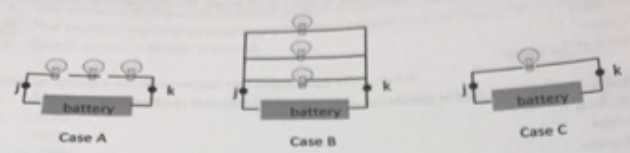# Problem: From lowest to highest, rank the overall value of the resistance across the circuit from the point labeled j to the point labeled k. a. A, B, C b. A, C, B c. B, A, C d. B, C, A e. C, A, B f. C, B, A

###### FREE Expert Solution

The bulbs act as resistors.

Assuming that each bulb has a resistance of R, we can get the equivalent resistance for the different cases.

Remember,

Equivalent resistance for resistors in parallel:

$\overline{)\frac{\mathbf{1}}{{\mathbf{R}}_{\mathbf{eq}}}{\mathbf{=}}\frac{\mathbf{1}}{{\mathbf{R}}_{\mathbf{1}}}{\mathbf{+}}\frac{\mathbf{1}}{{\mathbf{R}}_{\mathbf{2}}}{\mathbf{+}}{\mathbf{.}}{\mathbf{.}}{\mathbf{.}}{\mathbf{+}}\frac{\mathbf{1}}{{\mathbf{R}}_{\mathbf{n}}}}$

Equivalent resistance for resistors in series:

$\overline{){{\mathbf{R}}}_{{\mathbf{eq}}}{\mathbf{=}}{{\mathbf{R}}}_{{\mathbf{1}}}{\mathbf{+}}{{\mathbf{R}}}_{{\mathbf{2}}}{\mathbf{+}}{\mathbf{.}}{\mathbf{.}}{\mathbf{.}}{\mathbf{+}}{{\mathbf{R}}}_{{\mathbf{n}}}}$

86% (285 ratings)###### Problem DetailsFrom lowest to highest, rank the overall value of the resistance across the circuit from the point labeled j to the point labeled k.

a. A, B, C

b. A, C, B

c. B, A, C

d. B, C, A

e. C, A, B

f. C, B, A

Frequently Asked Questions

What scientific concept do you need to know in order to solve this problem?

Our tutors have indicated that to solve this problem you will need to apply the Combining Resistors in Series & Parallel concept. You can view video lessons to learn Combining Resistors in Series & Parallel. Or if you need more Combining Resistors in Series & Parallel practice, you can also practice Combining Resistors in Series & Parallel practice problems.